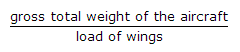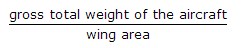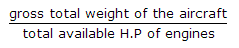# Online Civil Engineering Test - Airport Engineering TestLoading Test...

Instruction:

• This is a FREE online test. DO NOT pay money to anyone to attend this test.
• Total number of questions : 20.
• Time alloted : 30 minutes.
• Each question carry 1 mark, no negative marks.
• DO NOT refresh the page.
• All the best :-).

1.

The bearing of the longest line of a wind rose is S 45° E, the bearing of the runway will be numbered

A.
 135°B.
 315°C.
 13D.
 31E.
 both (c) and (d)2.

The lift off distance is the distance along the centre of the runway between the starting point and

A.
 end of the runwayB.
 end of stopwayC.
 point where air craft becomes air borneD.
 point where air craft attains a height of 10.7 mE.
 none of these.3.

According to I.C.A.O. the slope of transitional surface at right angles to the centre line of runway, is kept

A.
 1 in 4B.
 1 in 5C.
 1 in 6D.
 1 in 7E.
 1 in 104.

The depressions and undulations in the pavement, are caused due to

A.
 improper compaction of subgradeB.
 impact of heavy wheel loadsC.
 punching effectD.
 flexible gearsE.
 all the above.5.

Pick up the correct statement from the following:

A.
 The speed of the aircraft relative to the ground, is called cruising speedB.
 The speed of the aircraft relative to wind, is called air speedC.
 When wind is blowing the direction of the flight, air speed is less than cruising speedD.
 All the above.6.

Airport elevation is the reduced level above M.S.L. of

A.
 control towerB.
 highest point of the landing areaC.
 lowest point of the landing areaD.
 none of these.7.

Pick up the correct statement from the following:

A.
 The distance between the points of intersection of the extreme tangents to the transition curve is kept greater than 7500 m x sum of grade changes at the point of intersectionB.
 The rate of change of grade is limited to 0.3% per 30 m length of the curveC.
 According to I.C.A.O. the maximum longitudinal gradient along a runway is limited to 1.5%D.
 All the above.8.

The fuse large includes

A.
 passengers chamberB.
 pilot's cabinC.
 tail of aircraftD.
 all the above.9.

A.
 load of the wingsB.C.D.E.
 None of these.10.

Speed of

A.
 sound at 0°C is 1190 km p.h.B.
 sound varies 2.4 km p.h. per degree centrigrade rise in temperatureC.
 sound at 0°C is called one MachD.
 an aircraft flying at 2975 km p.h., is called 2.5 MachE.
 All the above.11.

The bearing of the runway at threshold is 290°, the runway number is

A.
 N 70° WB.
 290°C.
 29°D.
 W 20° N12.

The best direction of a runway is along the direction of

A.
 longest line on wind rose diagramB.
 shortest line on the wind rose diagramC.
 line clear of wind rose diagramD.
 none of these.13.

The landing and take off of the air craft is made against the direction of wind. In no case the centre line of the runway should make an angle with the wind direction exceeding

A.
 10°B.
 20°C.
 30°D.
 40°14.

According to I.C.A.O. all markings on the runways are painted white and on taxiways

A.
 blackB.
 redC.
 yellowD.
 green15.

Pick up the correct statement from the following:

A.
 Minimum turning radius of aircrafts decides the size of the apron and the radius of the curves at taxi-endsB.
 Take off and landing distances for an aircraft, determine the minimum runway lengthC.
 The length of the normal haul of the air craft decides the frequency of operationD.
 All the above.16.

Total correction for elevation, temperature and gradient for a runway should not be more than

A.
 15%B.
 20%C.
 25%D.
 30%E.
 35%17.

Two single runways may be arranged so as to have

A.
 L-shapeB.
 T-shapeC.
 X-shapeD.
 All the above.18.

The length of runway is increased per 300 m rise above M.S.L.

A.
 3%B.
 4%C.
 5%D.
 6%E.
 7%19.

Pick up the correct statement from the following:

A.
 Tar concrete pavements are suitable if fuel spillage occursB.
 Rubberised tar concrete hot blast as well as spillageC.
 Epoxy asphalt concrete resists the jet fuels and provides more elastic pavementD.
 Epoxy asphalt concrete sets in very small timeE.
 All the above.20.

The length of a runway under standard atmospheric conditions is 1800 m. If the actual reduced level of the site is 1200 m, the design length of the runway is

A.
 2360 mB.
 2460 mC.
 2560 mD.
 2660 m